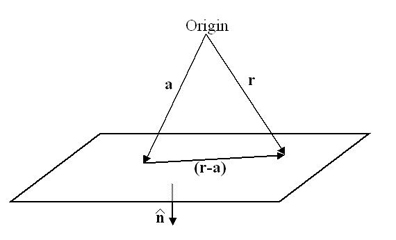# 8.4: The general case in three dimensions

$$\newcommand{\vecs}{\overset { \rightharpoonup} {\mathbf{#1}} }$$ $$\newcommand{\vecd}{\overset{-\!-\!\rightharpoonup}{\vphantom{a}\smash {#1}}}$$$$\newcommand{\id}{\mathrm{id}}$$ $$\newcommand{\Span}{\mathrm{span}}$$ $$\newcommand{\kernel}{\mathrm{null}\,}$$ $$\newcommand{\range}{\mathrm{range}\,}$$ $$\newcommand{\RealPart}{\mathrm{Re}}$$ $$\newcommand{\ImaginaryPart}{\mathrm{Im}}$$ $$\newcommand{\Argument}{\mathrm{Arg}}$$ $$\newcommand{\norm}{\| #1 \|}$$ $$\newcommand{\inner}{\langle #1, #2 \rangle}$$ $$\newcommand{\Span}{\mathrm{span}}$$ $$\newcommand{\id}{\mathrm{id}}$$ $$\newcommand{\Span}{\mathrm{span}}$$ $$\newcommand{\kernel}{\mathrm{null}\,}$$ $$\newcommand{\range}{\mathrm{range}\,}$$ $$\newcommand{\RealPart}{\mathrm{Re}}$$ $$\newcommand{\ImaginaryPart}{\mathrm{Im}}$$ $$\newcommand{\Argument}{\mathrm{Arg}}$$ $$\newcommand{\norm}{\| #1 \|}$$ $$\newcommand{\inner}{\langle #1, #2 \rangle}$$ $$\newcommand{\Span}{\mathrm{span}}$$$$\newcommand{\AA}{\unicode[.8,0]{x212B}}$$

After considering these simple examples, it should hopefully be clear how to construct Brillouin Zones. Whilst in two dimensions this geometric method is easy to apply, in three dimensions the lattice cannot be represented on a piece of paper and in general it is much harder to picture the shape of the Brillouin Zones beyond the first. This section considers how the relevant Bragg planes for zone construction may be generated in a systematic fashion.

In vector notation, the equation of a plane may be written as

$(\mathbf{r}-\mathbf{a}) \cdot \hat{\mathbf{n}}=0$

In this expression, a is a vector from the origin to a specified (but arbitrary) point in the plane, r is a general point in the plane and $$\hat{\mathbf{n}}$$ is the unit vector normal to the plane.For Brillouin Zones it is convenient to choose a so that it is the perpendicular vector from the origin to the Bragg plane of interest, i.e., a vector of the form

$\mathbf{a}=\frac{1}{2}\left(h \mathbf{b}_{1}+k \mathbf{b}_{2}+l \mathbf{b}_{3}\right)$

also, the unit normal is then given by

$\hat{\mathbf{n}}=\frac{\mathbf{n}}{|\mathbf{a}|}$

Letting h, k and l be integers (positive or negative) and excluding the point where all three are equal to zero, the relevant Bragg Planes may be generated in a systematic fashion. It is usually sufficient for finding the first three or four zones to have the di range between –3 and +3.

Then, given any point in reciprocal space, it may be allocated to a Brillouin zone by determining the number, N, of Bragg Planes that lie in between that point and the origin. The point is then in the (N+1)th Brillouin Zone.

Whether a Bragg plane lies between a general point, r, and the origin may be determined quite simply by considering the projection of the position vector of the point in the direction of the unit normal to the Bragg plane. The scalar product

$\mathbf{a} \cdot(\mathbf{r}-\mathbf{a})$

is positive if the plane is between the point and the origin, and is negative when the plane does not lie between the point and the origin.

The main complication when extending to Brillouin Zones beyond the first zone is that it is very easy to overlook an important Bragg Plane. This can only really be avoided by being careful and systematic. A useful test to indicate if a mistake has been made is to check that all the zones have the same symmetries as the reciprocal lattice itself. For example the Brillouin Zones for the 2-D Square lattice always have fourfold-rotational symmetry about the origin. A further useful check is to confirm that the area (in 2D) or volume (in 3D) of each Brillouin zone is the same.

Some examples which show the Brillouin Zones for common 2-D lattices may be found by clicking the links below.

This page titled 8.4: The general case in three dimensions is shared under a CC BY-NC-SA license and was authored, remixed, and/or curated by Dissemination of IT for the Promotion of Materials Science (DoITPoMS).